### Home > PC > Chapter 1 > Lesson 1.2.2 > Problem1-83

1-83.
1.Write each expression in a simpler form by using the smallest base. (The answer will not always be in pq form.) Homework Help ✎

1. 62 · 36−3 · 2160

2.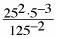3. (3 · 1997) ÷ (8 · 1994)

4. (3 · 19−97) ÷ (8 · 19−94)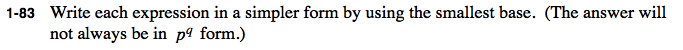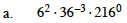$216\degree=1$

$6^{-4}$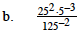Convert all the bases to 5.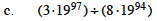$\frac{3\cdot19^{97}}{8\cdot 19^{94}}=$

$\frac{3}{8} \cdot 19^{97-94}=$

$\frac{3}{8} \cdot 19^3$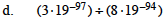Follow the same process as (c).